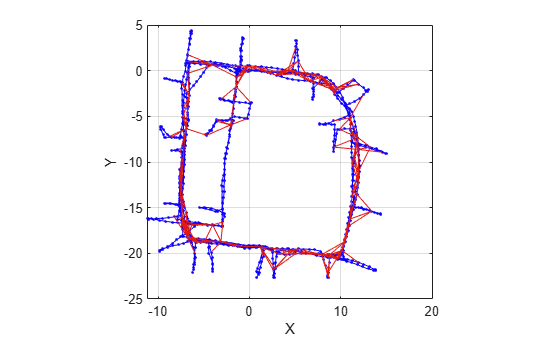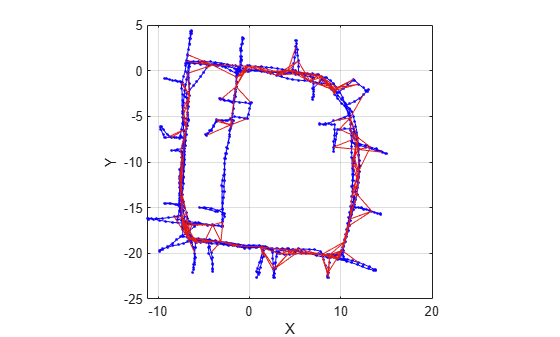# findEdgeID

Find edge ID of edge

Since R2019b

## Syntax

``edgeID = findEdgeID(poseGraph,nodePairs)``

## Description

example

````edgeID = findEdgeID(poseGraph,nodePairs)` finds the edge ID for a specified edge. Edges are defined by the IDs of the two nodes that connect them.```

## Examples

collapse all

Load the Intel Research Lab Dataset that contains a 2-D pose graph. Optimize the pose graph. Plot the pose graph with IDs off. Red lines indicate loop closures identified in the dataset.

```load intel-2d-posegraph.mat pg optimizedPG = optimizePoseGraph(pg); show(optimizedPG,IDs="off");```Find the loop closure edge ID for the specified edge node pair in pose graph.

```nodePair = [133 1085]; edgeID = findEdgeID(optimizedPG,nodePair)```
```edgeID = 1386 ```

Remove the loop closure edge from the pose graph.

```removeEdges(optimizedPG,edgeID) show(optimizedPG,IDs="off");```## Input Arguments

collapse all

Pose graph, specified as a `poseGraph` or `poseGraph3D` object.

Edge node pairs in pose graph, specified as a two-element vector that lists the IDs of the two nodes that the edge connects.

## Output Arguments

collapse all

Edge IDs, returned as a positive integer or vector of positive integers. The pose graph can contain multiple edges between each node pair, so multiple edge IDs may be returned for a single edge ID.

## Version History

Introduced in R2019b